# 3.2.8. Molecule Plot¶

The Molecule plot takes as input data with atoms and bonds (stored internally as Vertices and Lines in a VTK PolyData structure) and renders it as spheres and lines/cylinders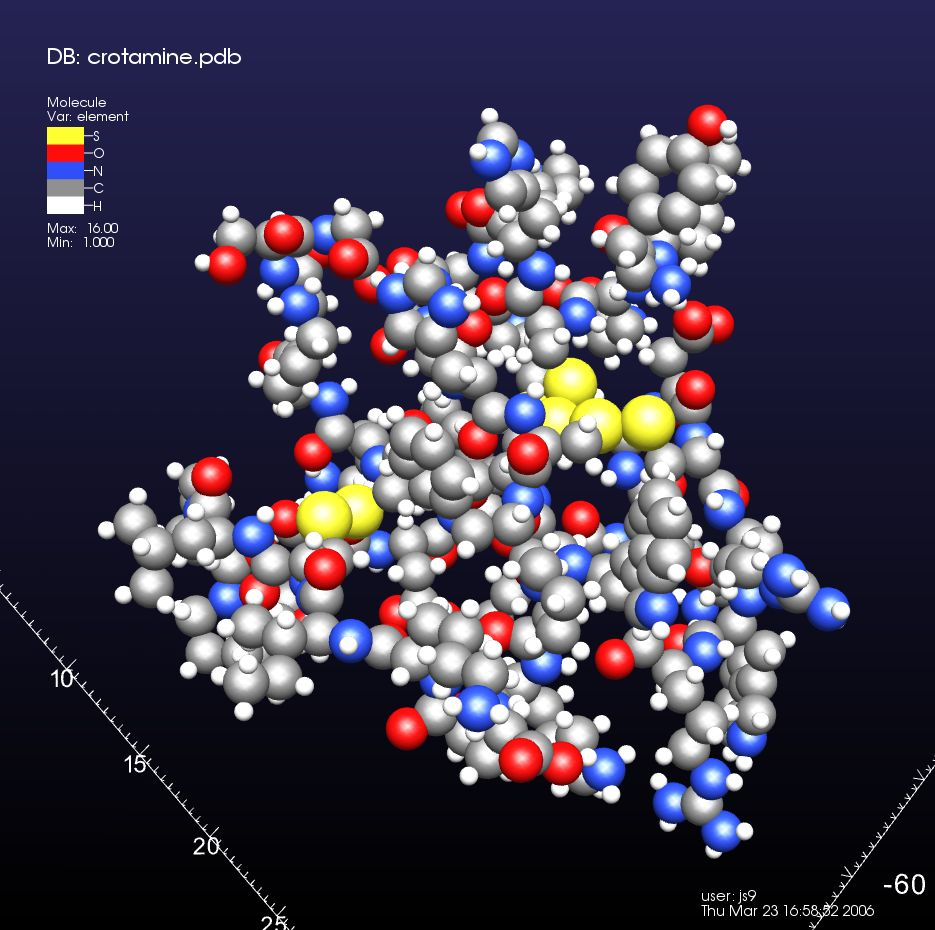Fig. 3.33 Molecule plot of crotamine, colored by element type, atoms shown with covalent radius, and no bonds.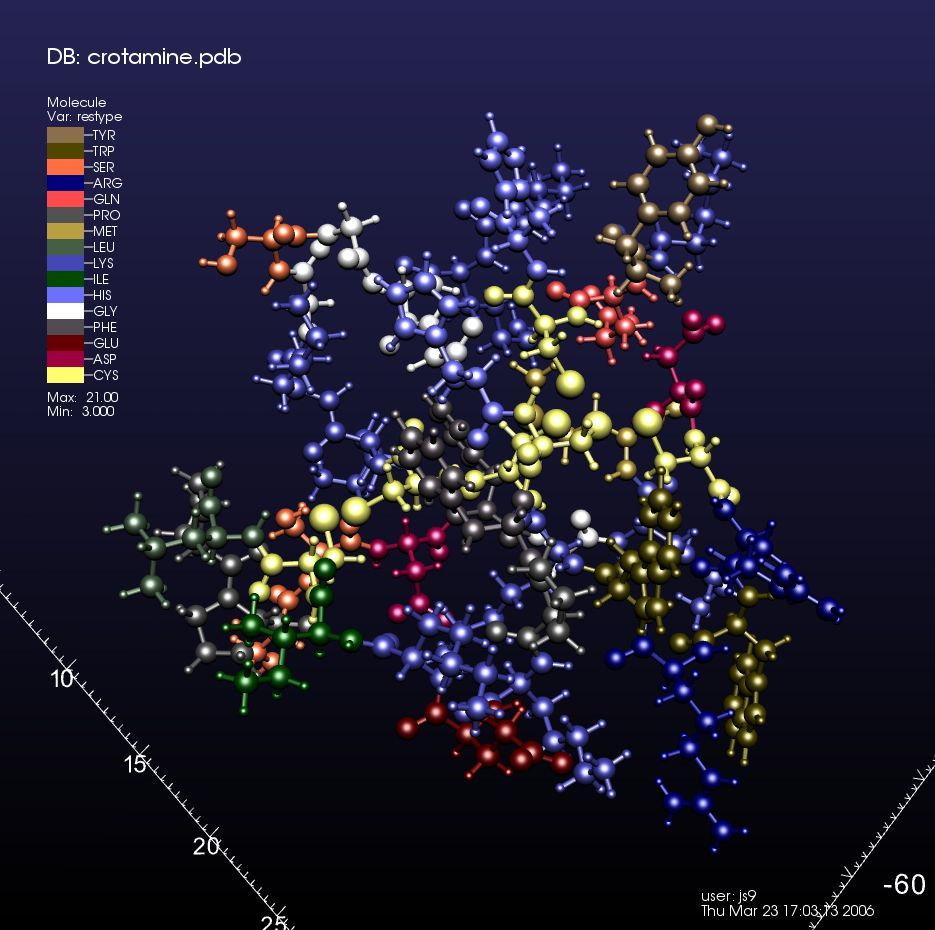Fig. 3.34 Molecule plot of crotamine, colored by residue type, atoms proportional to covalent radius emulating the CPK syle, bonds colored with adjacent atom color.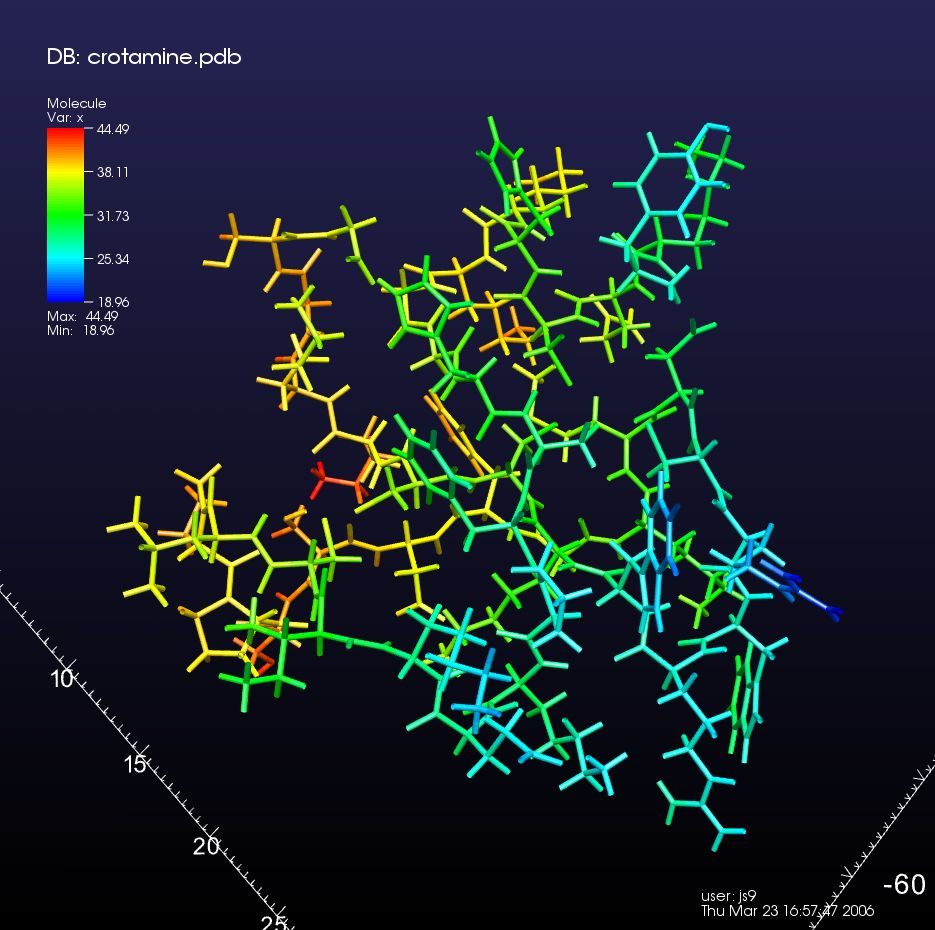Fig. 3.35 Molecule plot of crotamine, colored by a scalar quantity, no atoms shown, and bonds drawn as cylinders.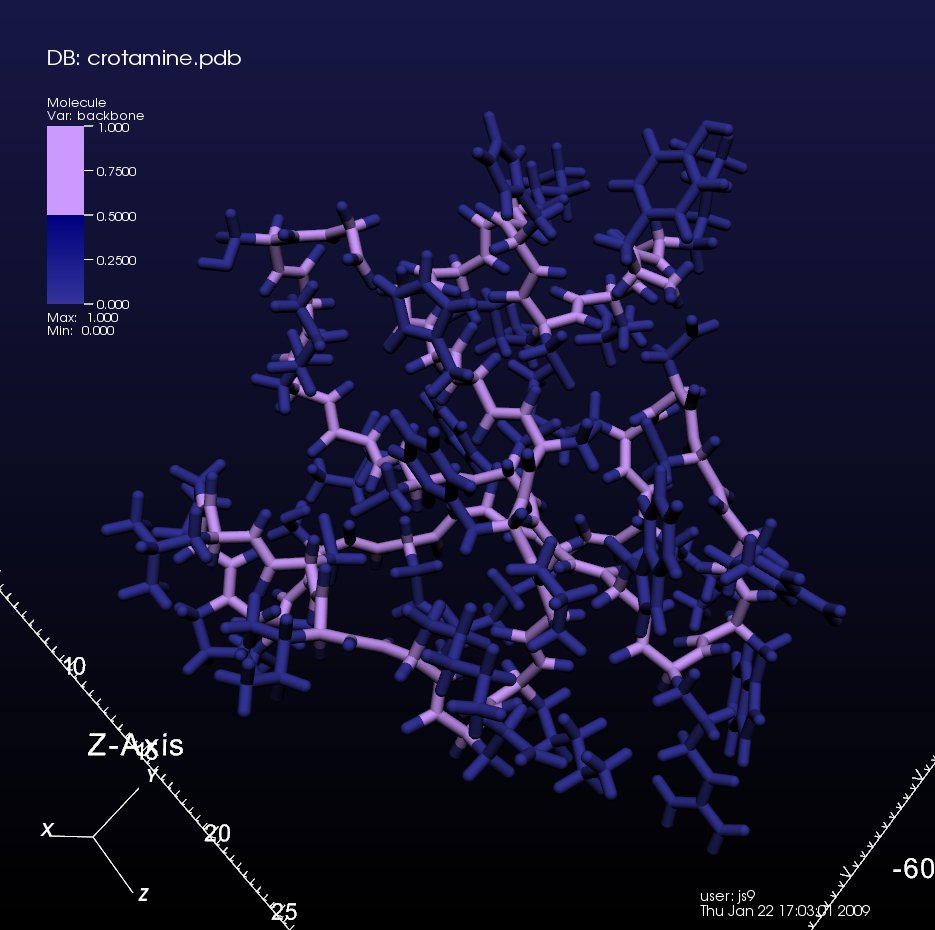Fig. 3.36 Molecule plot of crotamine, colored by backbone, atom at same width as thicker cylinder-shaped bonds.

## 3.2.8.1. Controlling how atoms are drawn¶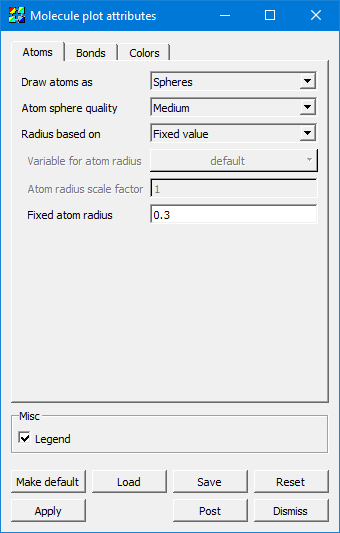Fig. 3.37 Molecule plot window Atoms tab

The value Spheres for Draw atoms as means to draw spheres using 3D geometry. Sphere Impostors means to draw them using a single flat polygon with an image of a sphere – this requires support from graphics hardware and can introduce some minor graphical artifacts, but it is very fast. The value None means that you are only interested in seeing the bonds, and you would like the atoms themselves not to be drawn.

When rendering Spheres, Atom sphere quality determines the number of polygons used to draw the atom geometry. Low corresponds to about a dozen polygons per sphere, Medium is several dozen, High a couple hundred, and Super is about a thousand.

Radius based on determines how the atoms are sized. Scalar variable uses a nodal variable on the data set to determine radius. Covalent radius and Atomic radius are the atomic properties, and they are calculated using a built-in lookup table in VisIt. Fixed value simply uses the value in the text field below as the radius. Note that Covalent radius and Atomic radius require a discrete nodal field called element to exist and contain the atomic number. Also, note that some default values are set due to much molecular data being in units of Angstroms. Depending on your data, you may need to change the atomic/bond radii.

When Radius based on is set to Scalar variable, the Variable for atom radius field becomes active and determines which variable shall be used (and multiplied by the scale factor below) as the value for the radius of the rendered atoms.

Atom radius scale factor applies when Radius based on is not set to Fixed value. This value multiplies the other value used for radius, whether it is the atomic/covalent radius or based on a scalar variable. Note that the atomic and covalent radii used are in Angstroms, so if your data is in other units, you should apply the appropriate conversion factor here.

Fixed atom radius only applies when Radius based on is set to Fixed value. It is the actual radius you want to use to draw the atoms in world coordinate units.

## 3.2.8.2. Controlling how bonds are drawn¶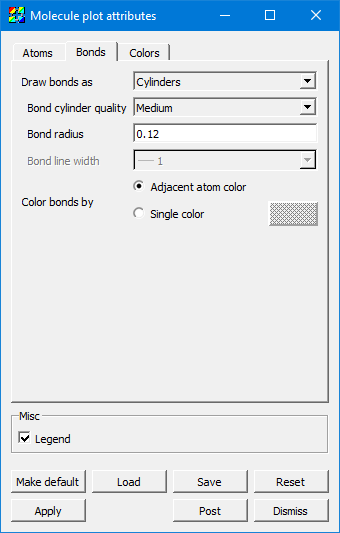Fig. 3.38 Molecule plot window Bonds tab

The value None for Draw bonds as means you are only interested in seeing the atoms and would like any bonds to be hidden. Lines uses geometric lines with no 3D shading, and Cylinders uses 3D geometry with 3D shading. Lines is much faster but Cylinders looks better.

When Draw bonds as is set to Cylinders, Bond cylinder quality determines the number of polygons used to draw the bonds. Low is about three polygons, and High is about twenty.

When Draw bonds as is set to Cylinders, Bond radius determines the thickness of the cylinder in world coordinate units. Note that defaults for these values were chosen due to molecular data commonly being in units of Angstroms. Depending on your data, you may need to change the radius used for rendering atoms and bonds.

When Draw bonds as is set to Lines, Bond line width determines the thickness of the line used to draw the bonds in terms of a number of pixels.

Color bonds by can be set to Adjacent atom color, which means that each half of the bond is drawn using the color of the atom to which it is attached. Or, it can be set to a Single color chosen at the color selector just to the right of this checkbox.

## 3.2.8.3. Controlling colors¶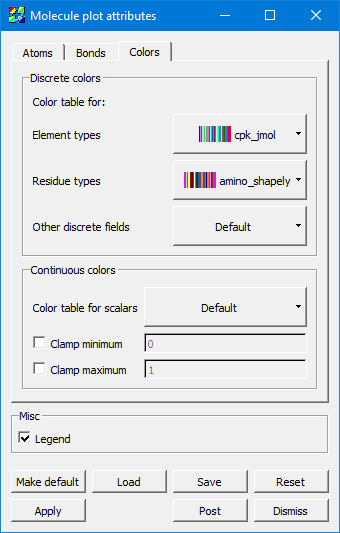Fig. 3.39 Molecule plot window Colors tab

The Discrete colors group is for values which take on integral values. When VisIt encounters a discrete-valued variable, it determines which one of these color tables to use based on the variable name (element and restype, specifically).

Element types and Residue types are specific examples, and they are separate because there are conventional color tables widely used. VisIt provides some of these color tables. Other discrete fields catches anything which is not an element or residue type.

The Continuous colors group is for values which take on real values. Color table for scalars can be set to any color table, typically a continuous one. The Clamp minimum and Clamp maximum check boxes, along with their values, toggle whether to clamp the continuous field to narrow the range to a specific range of values of particular interest, making full use of the color table within that range and clamping anything outside that range to the colors at the min/max extrema of the selected color table.

## 3.2.8.4. Examples in use¶

See Molecular data features for examples of the Molecule plot in use.